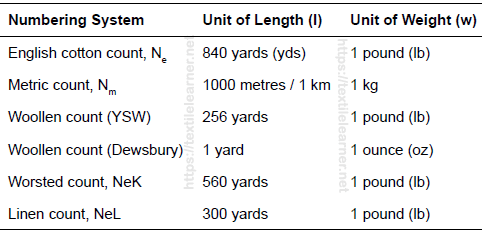# Understanding Yarn Count | Yarn Counting System

Last Updated on 04/06/2021

### Understanding Yarn Count | Yarn Counting System

Mustaque Ahammed Mamun
Department of Textile Engineering
Dhaka University of Engineering & Technology (DUET)
Email: mamuntex09@gmail.com

Linear Density or Count:
Yarn count is a numerical value which expresses the coarseness or fineness (diameter) of the yarn and also indicates the relationship between length and weight (the mass per unit length or the length per unit mass) of that yarn. The fineness of the yarn is usually expressed in terms of its linear density or count. If you see the below figure, concept of yarn count will be clear.

According to Textile Institute, “Count is a number indicating the mass per unit length or the length per mass unit of a yarn”.

Types of Yarn Counting System
There are two types of yarn counting system:

1. Direct system
2. Indirect system

1. Direct system: Yarn count is measured as the weight of yarn per unit length. The common feature of all direct count systems is the length of yarn fixed, and the weight of yarn varies according to its fineness. Heavier yarn has greater weight per unit length. Traditionally, the direct system is used in the metric system.

In this yarn counting system, If Count increase then Dia increase.

………………….Weight
Count = ———————————-
……………..Unit Length

For Direct system Used formula,

………W x l
N = ——————
………w x L

Where,

N = count
L = sample length
l = unit length
W = wt. of yarn
w = wt. unit

Direct yarn count system:a. Denier system
Weight in grams of yarn/fiber of 9000 meter length is called the Denier of that particular yarn/fiber.

Denier = weight in grams of 9000 meters length

b. Tex System
Weight in grams of yarn/fiber of 1000 meter length is called the Tex of that particular yarn/fiber.

Tex = weight in grams of 1000 meters length

2. Indirect system: Count is measured as the length of yarn per unit weight. The common feature of all indirect count systems is the weight of yarn fixed, and the length of yarn varies according to its fineness. Less heavy yarn has greater length per unit weight. Indirect system is used in the English system.

In this yarn counting system, If increase Count then decrease Dia.

………………….Length
Count = —————————–
………………Unit Weight

For Indirect system Used formula,

……….L x w
N = ——————–
……….l x W

Where,

N = count
L = sample length
l = unit length
W = wt. of yarn
w = wt. unit

Indirect yarn count system:Types of yarn count:

1. Normal count
2. Conditional count
3. Average count
4. Resultant count

Relation between yarn diameter and count:

Assuming an apparent specific volume for cotton yarn is 1.1:

Specific volume of yarn is 1.1 cc/gm

The mass of 1.1 cc yarn = 1 gm

If the yarn count is N tex then, we get

The length of N gm yarn is 1000m

The length of 1 gm yarn is 1000/N m=100000/N m

So that, length of 1.1 cc yarn is 100000/N m

We know that,
Cross sectional area × Length = Volume

If yarn Diameter is d then,

……..d2        105
π x ——- x ——— = 1.1
……..4           N

…….0.375
d = ———–√N cm (For direct system)
……..100

Converting cm to inches and Tex to English count,

…………1
d = ————— inch (For indirect system only)
……..28√NE

Measurement of Yarn Counting System:

1. Wrap reel and balance method
2. Beesley’s yarn balance
3. Knowles balance